Inverse Proportion

Chapter 11 Class 8 Direct and Inverse Proportions
Concept wise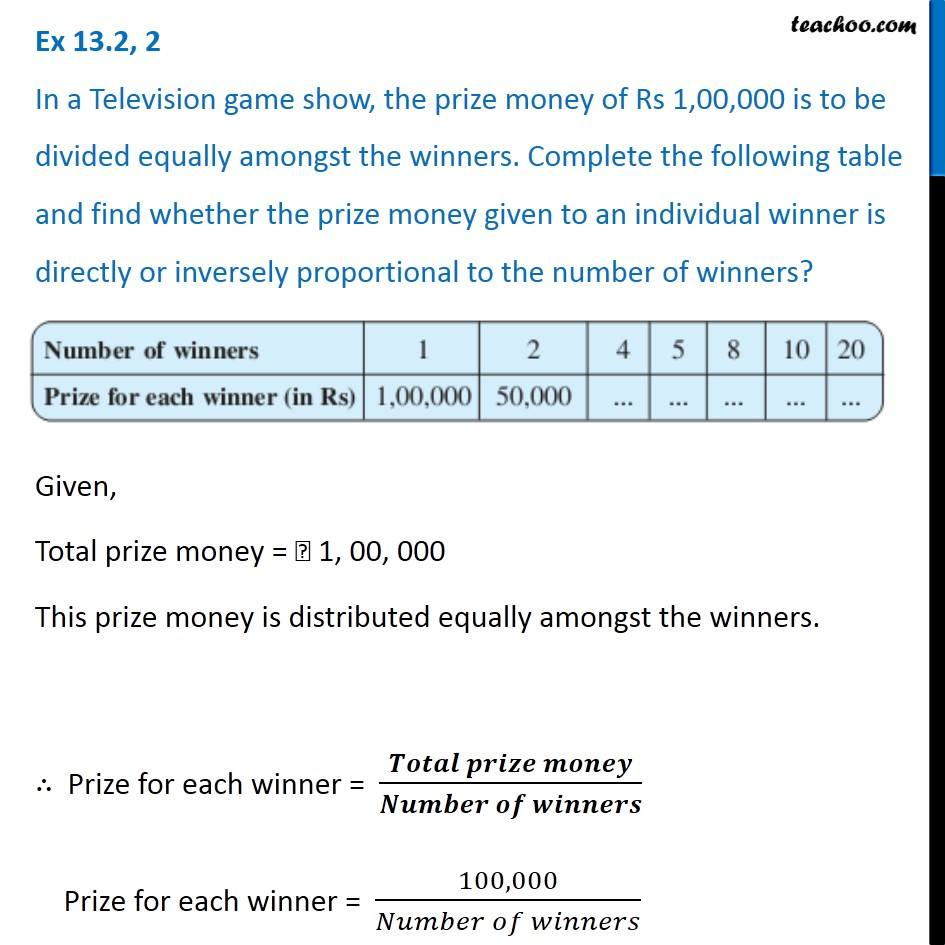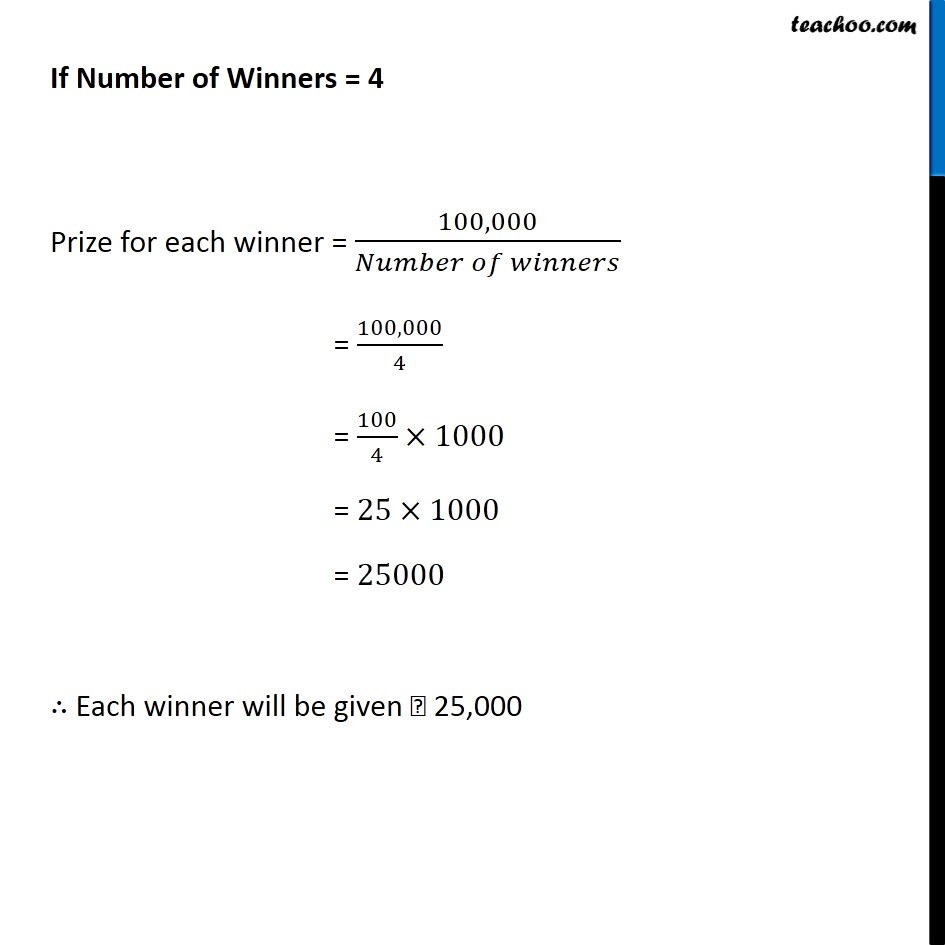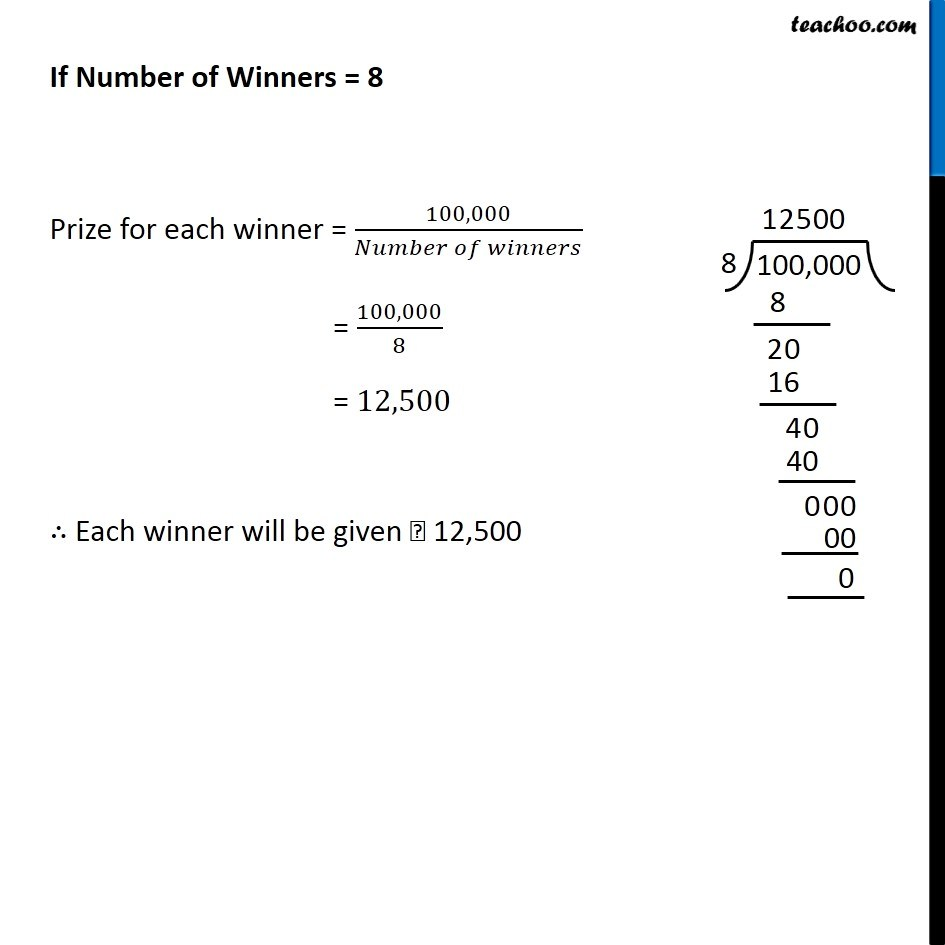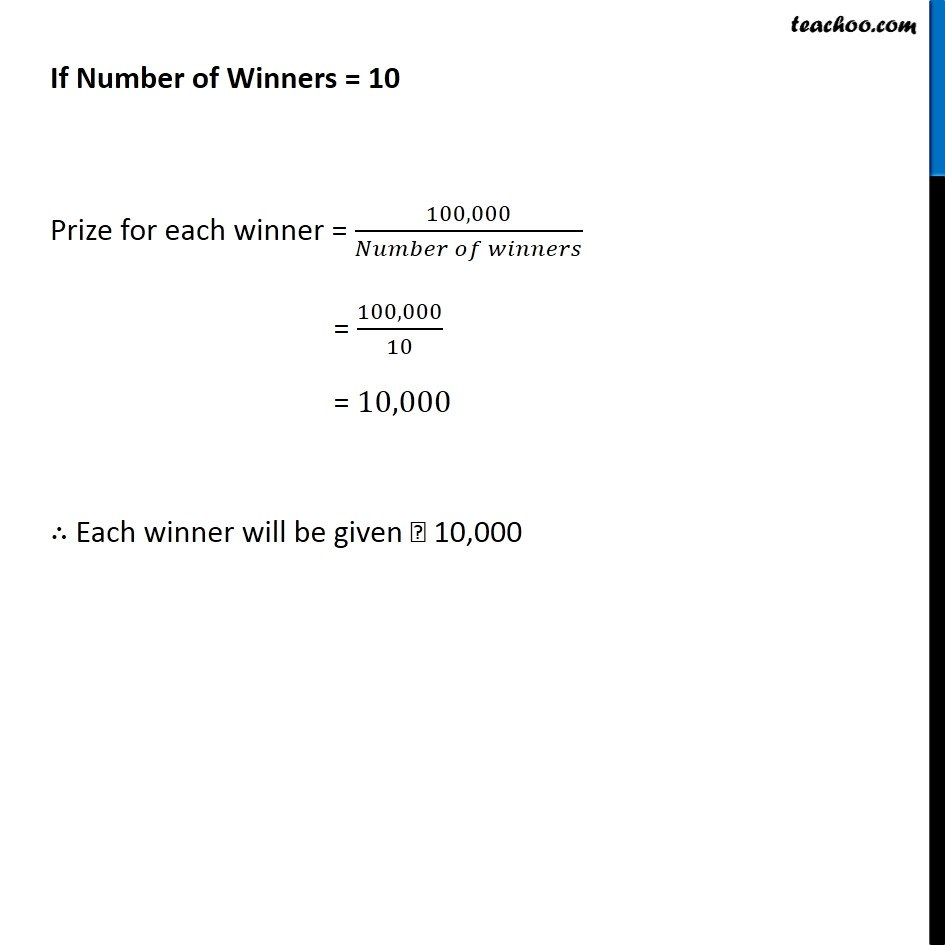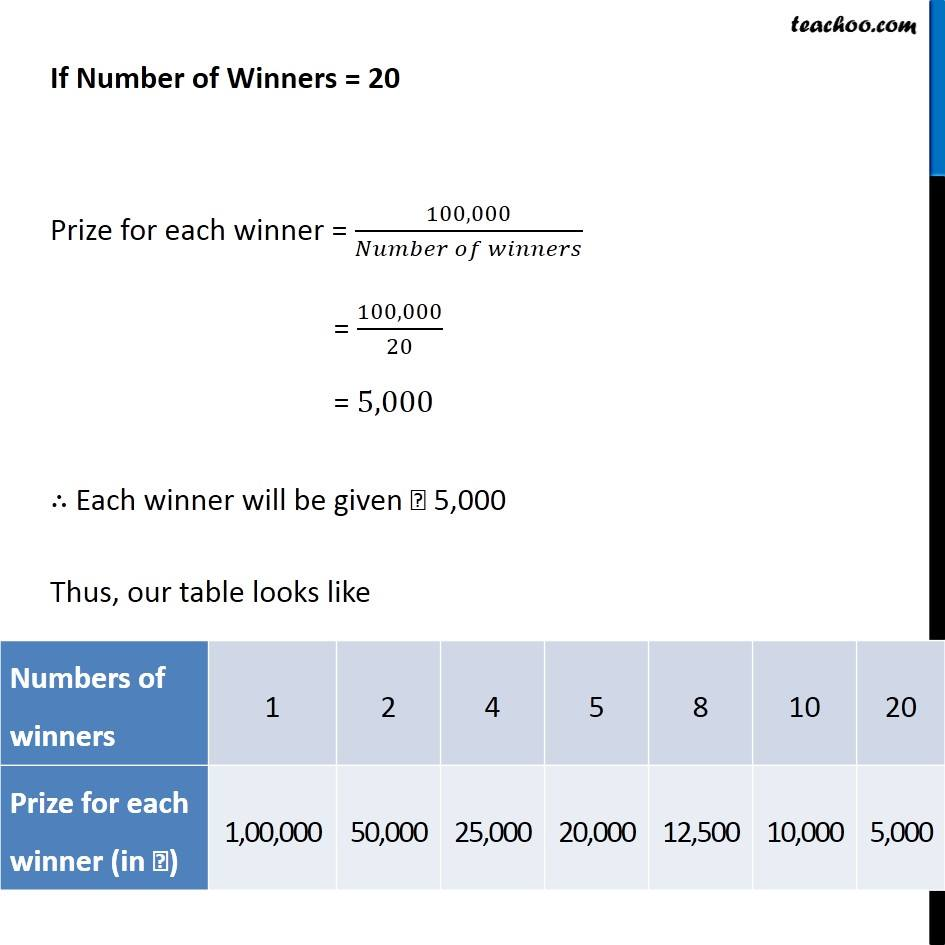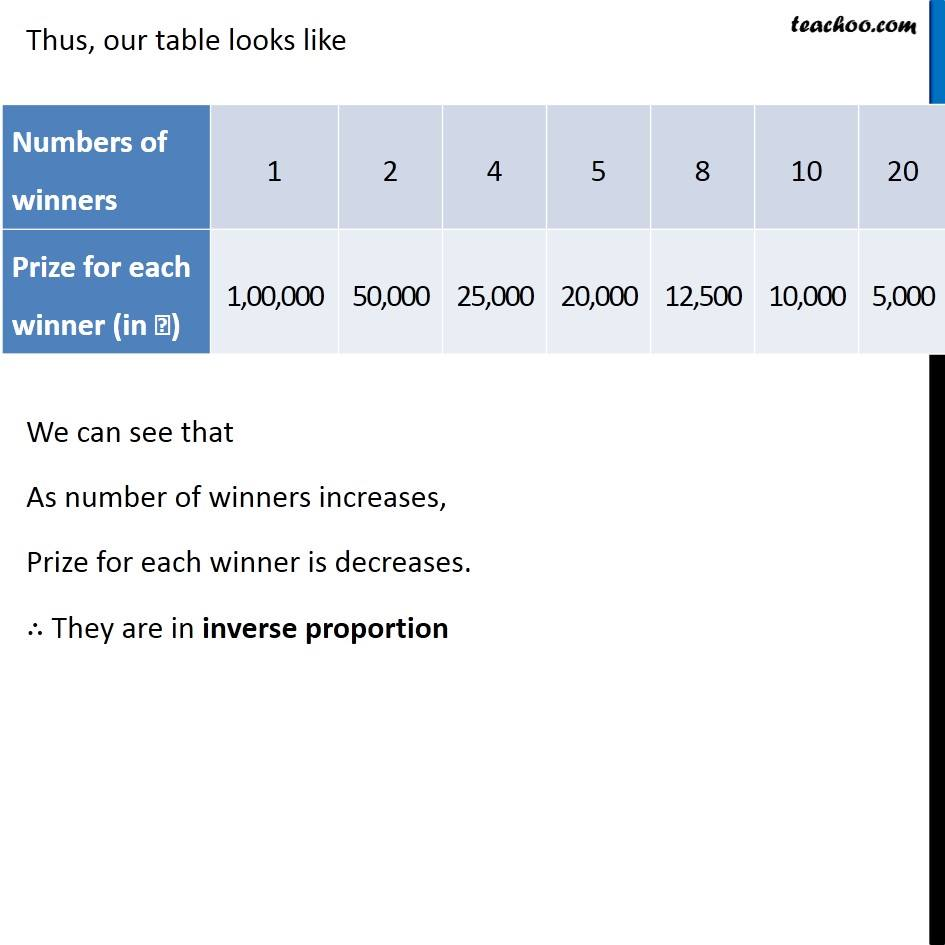Learn in your speed, with individual attention - Teachoo Maths 1-on-1 Class

### Transcript

Ex 11.2, 2 In a Television game show, the prize money of Rs 1,00,000 is to be divided equally amongst the winners. Complete the following table and find whether the prize money given to an individual winner is directly or inversely proportional to the number of winners? Given, Total prize money = ₹ 1, 00, 000 This prize money is distributed equally amongst the winners. ∴ Prize for each winner = (𝑻𝒐𝒕𝒂𝒍 𝒑𝒓𝒊𝒛𝒆 𝒎𝒐𝒏𝒆𝒚)/(𝑵𝒖𝒎𝒃𝒆𝒓 𝒐𝒇 𝒘𝒊𝒏𝒏𝒆𝒓𝒔) Prize for each winner = (100, 000)/(𝑁𝑢𝑚𝑏𝑒𝑟 𝑜𝑓 𝑤𝑖𝑛𝑛𝑒𝑟𝑠) If Number of Winners = 4 Prize for each winner = (100, 000)/(𝑁𝑢𝑚𝑏𝑒𝑟 𝑜𝑓 𝑤𝑖𝑛𝑛𝑒𝑟𝑠) = (100, 000)/4 = 100/4×1000 = 25×1000 = 25000 ∴ Each winner will be given ₹ 25,000 If Number of Winners = 8 Prize for each winner = (100, 000)/(𝑁𝑢𝑚𝑏𝑒𝑟 𝑜𝑓 𝑤𝑖𝑛𝑛𝑒𝑟𝑠) = (100, 000)/8 = 12,500 ∴ Each winner will be given ₹ 12,500 If Number of Winners = 10 Prize for each winner = (100, 000)/(𝑁𝑢𝑚𝑏𝑒𝑟 𝑜𝑓 𝑤𝑖𝑛𝑛𝑒𝑟𝑠) = (100, 000)/10 = 10,000 ∴ Each winner will be given ₹ 10,000 If Number of Winners = 20 Prize for each winner = (100, 000)/(𝑁𝑢𝑚𝑏𝑒𝑟 𝑜𝑓 𝑤𝑖𝑛𝑛𝑒𝑟𝑠) = (100, 000)/20 = 5,000 ∴ Each winner will be given ₹ 5,000 Thus, our table looks like Thus, our table looks like We can see that As number of winners increases, Prize for each winner is decreases. ∴ They are in inverse proportion